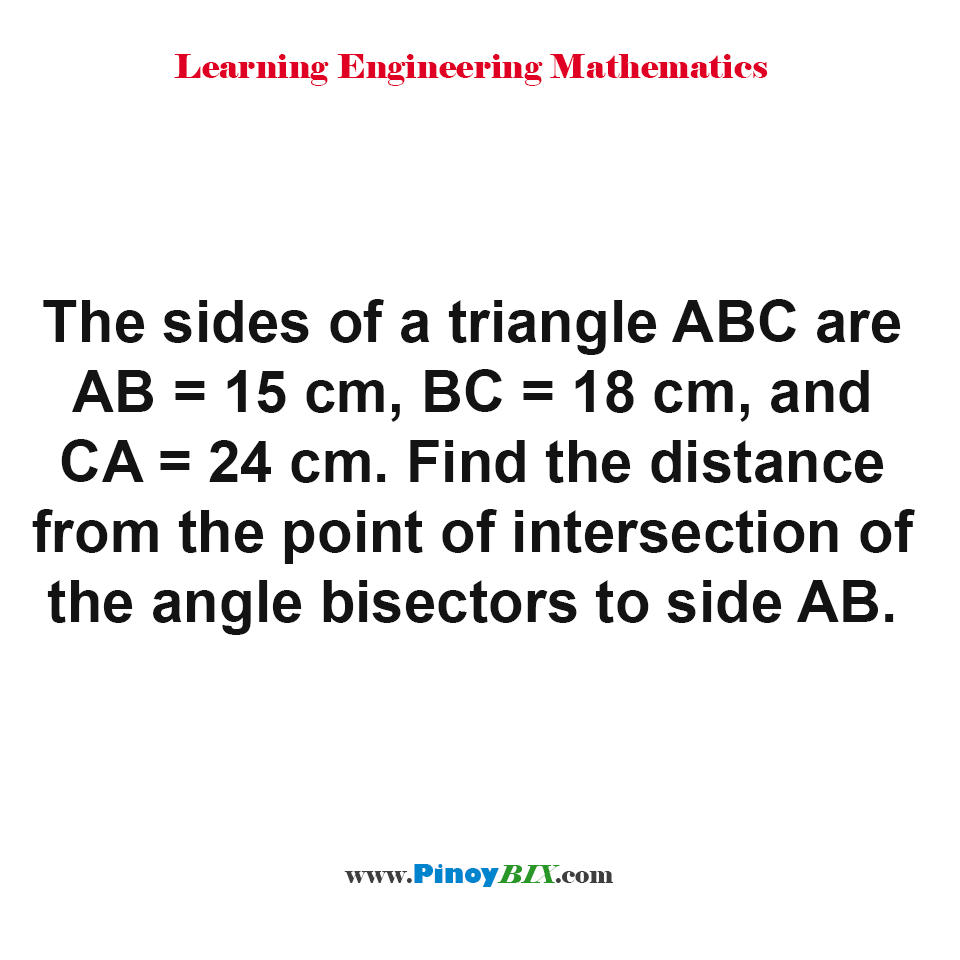# Solution: Find the distance from the point of intersection of the angle bisectors

(Last Updated On: January 25, 2020)#### Problem Statement:

The sides of a triangle ABC are AB = 15 cm, BC = 18 cm, and CA = 24 cm. Find the distance from the point of intersection of the angle bisectors to side AB.

#### Problem Answer:

The distance from the point of intersection of the angle bisectors to side AB is 4.73 cm. Note that by definition the point of intersection of all angle bisectors of a triangle is called incenter. It is also defined as the center of the inscribed circle of the triangle. Thus, the distance from incenter of the triangle to any of its sides is equal to the radius of the inscribed circle.

#### Latest Problem Solving in Plane Trigonometry Problems

More Questions in: Plane Trigonometry Problems

#### Online Questions and Answers in Plane Trigonometry Problems

Please do Subscribe on YouTube!

P inoyBIX educates thousands of reviewers and students a day in preparation for their board examinations. Also provides professionals with materials for their lectures and practice exams. Help me go forward with the same spirit.

“Will you subscribe today via YOUTUBE?”

Subscribe